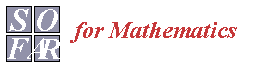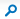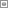# Blog

 This Site: BlogMathsShare > Blog > Posts > Mirror a point in a line - Oxford Mathematics entrance question

## BlogOctober September August Julyshow more ›
 There are no items in this list.September 17
 Mirror a point in a line - Oxford Mathematics entrance question

The normal to the line ax + by = c is the vector (a,b).
Hence the normal to the line 3x + 4y = 50 is (3,4).

Any point from (x1,y1) on a normal is of the form (x1,y1) + k(a,b) – which is, if I remember rightly, (3,4) + k(3,4) in the example question.  I think only (9,12) on the list of possible answers will fit? (with k=2).